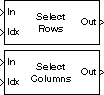# Variable Selector

Select subset of rows or columns from input

• Library:
• DSP System Toolbox / Signal Management / Indexing

•## Description

The Variable Selector block extracts a subset of rows or columns from the M-by-N input matrix `u` at each input port. You specify the number of input and output ports in the Number of input signals parameter.

When an element of the indexing vector references a nonexistent row or column of the input, the block implements the action that you specify by using the Invalid index parameter.

When the indexing vector elements are of the Boolean data type, the block performs logical indexing. Select Fill empty spaces in outputs (for logical indexing) to access the Fill values parameter. These values are appended to the output to make it as long as the input elements.

### Note

The Variable Selector block always copies the selected input rows or columns to a contiguous block of memory (unlike the Simulink® Selector block).

## Ports

### Input

expand all

Nth input signal, where N is the number of inputs specified in the Number of input signals parameter.

When the input is an unoriented vector, the Select parameter is ignored and the output is an unoriented vector of length L containing those elements specified by the length-L indexing vector.

Data Types: `single` | `double` | `int8` | `int16` | `int32` | `int64` | `uint8` | `uint16` | `uint32` | `uint64` | `Boolean` | `fixed point` | `enumerated`
Complex Number Support: Yes

Specify a scalar value or vector containing the indices of the input rows or columns that appear in the output matrix.

#### Dependencies

This port appears only when you set the Selector mode to `Variable`.

Data Types: `single` | `double` | `int8` | `int16` | `int32` | `int64` | `uint8` | `uint16` | `uint32` | `uint64` | `Boolean` | `fixed point` | `enumerated`

### Output

expand all

Nth output signal, corresponding to the Nth input signal, where N is the number of inputs specified in the Number of input signals parameter. The output is returned as a scalar, vector, or matrix as the same type, size, and complexity as the corresponding input.

For variable and fixed indexing modes, the row selection operation is equivalent to

`y = u(idx,:) % Equivalent MATLAB code `

and the column selection operation is equivalent to

`y = u(:,idx) % Equivalent MATLAB code `

where `idx` is the length-L indexing vector. The row selection output size is L-by-N and the column selection output size is M-by-L. Input rows or columns can appear any number of times in the output, or not at all.

Data Types: `single` | `double` | `int8` | `int16` | `int32` | `int64` | `uint8` | `uint16` | `uint32` | `uint64` | `Boolean` | `fixed point` | `enumerated`

## Parameters

expand all

Specify the number of input signals as a positive integer. An input port is created on the block for each input signal.

Specify the dimension of the input to select, `Rows` or `Columns`.

When Select is set to:

• `Rows` –– The block extracts rows from each input matrix.

• `Columns` –– The block extracts columns from each input matrix.

When the input is an unoriented vector, the Select parameter is ignored. The output is an unoriented vector of length L containing those elements specified by the length-L indexing vector.

Specify the type of indexing operation to perform, `Variable` or `Fixed`.

When Selector mode is set to:

• `Variable` –– The length-L vector input to the Idx port selects L rows or columns of each input to pass through to the output. You can update the elements of the indexing vector at each sample time, but the vector length must remain the same throughout the simulation.

• `Fixed` –– The Idx port is disabled. The length-L vector that you specified in the Elements parameter selects L rows or columns of each input to pass through to the output. The Elements parameter is tunable, so you can change the values of the indexing vector elements at any time during the simulation but the vector length must remain the same.

Specify a vector containing the indices of the input rows or columns that appear in the output matrix.

Tunable: Yes

#### Dependencies

This parameter appears only when you set the Selector mode to `Fixed`.

When set to `One-based`, an index value of `1` refers to the first row or column of the input. When set to `Zero-based`, an index value of `0` refers to the first row or column of the input.

Specify how the block handles an invalid index value. You can select one of these options:

• `Clip index` — Clip the index to the nearest valid value and do not issue an alert.

For example, if the block receives a `64`-by-`4` input and the Select parameter is set to:

• `Rows` –– The block clips an index of `72` to `64`.

• `Columns` –– The block clips an index of `72` to `4`.

In both cases, the block clips an index of `-2` to `1`.

• `Clip and warn` — Clip the index to the nearest valid value and display a warning message at the MATLAB® command line.

• `Generate error` — Display an error dialog box and terminate the simulation.

Tunable: Yes

When the indexing vector elements are of a Boolean data type, the block performs logical indexing. Logical indexing can cause empty spaces in the output. Select this parameter to designate values to be appended to the output in the Fill values parameter.

Specify the fill values when the block performs logical indexing.

#### Dependencies

This parameter appears only when you select the Fill empty spaces in outputs (for logical indexing) parameter.

## Block Characteristics

 Data Types `double` | `single` | `Boolean` | `base integer` | `fixed point` | `enumerated` Multidimensional Signals `No` Variable-Size Signals `No`